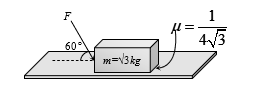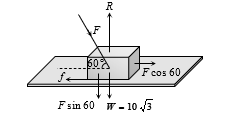Friction as component of Net Contact Force
Question

# What is the maximum value of the force F such that the block shown in the arrangement, does not moveEasy
Solution

##$\mathrm{f}=\mathrm{\mu R}$$\mathrm{Fcos}60°=\mathrm{\mu }\left(\mathrm{W}+\mathrm{Fsin}60°\right)$

Get Instant Solutions